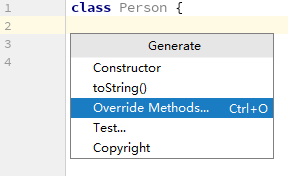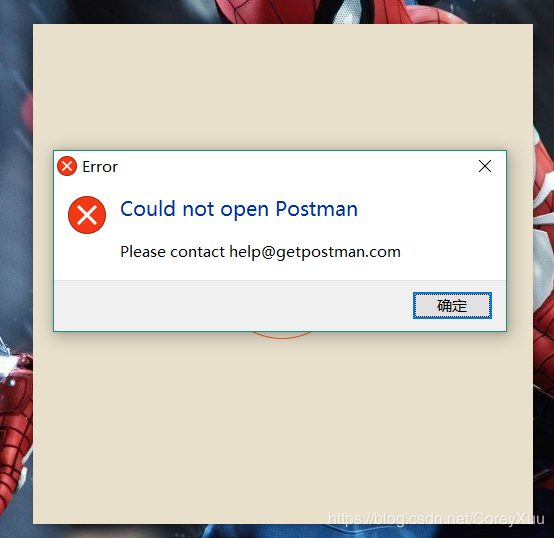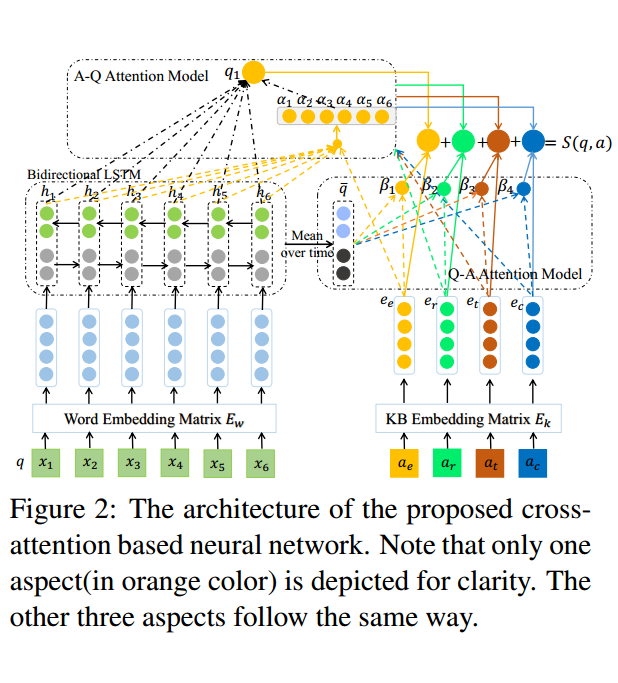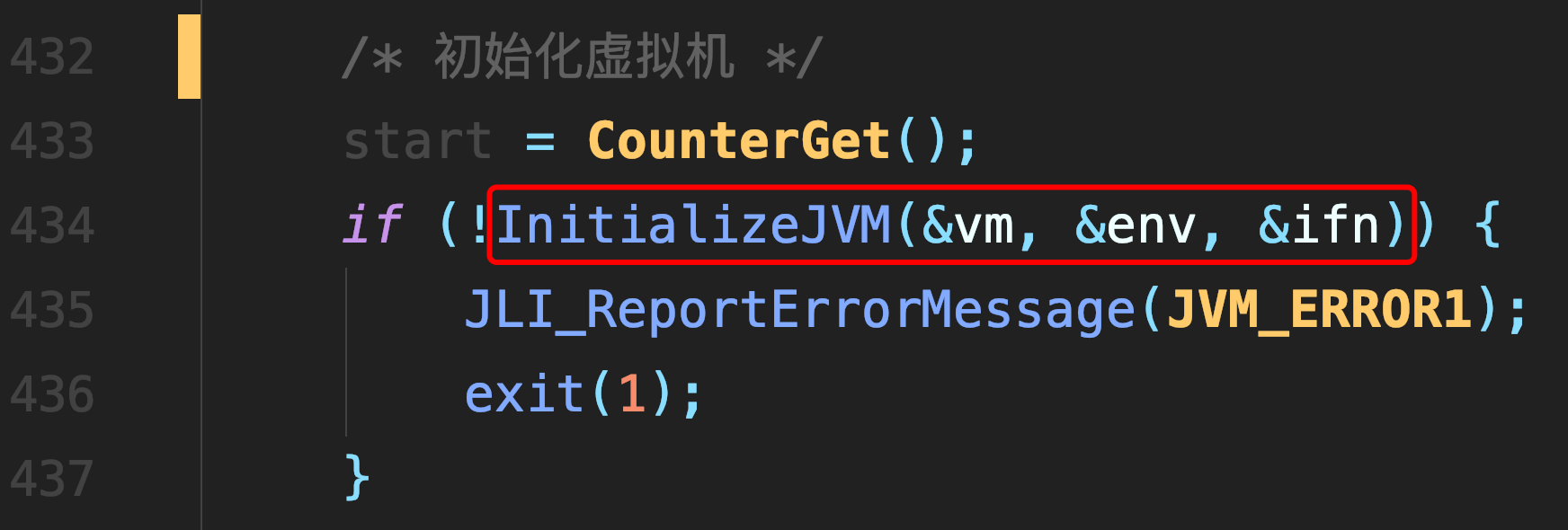# PCA(主成分分析法)详解

### PCA(主成分分析法)详解

PCA是一种经典的降维方法，采用组合特征的方法来进行降维。

PCA方法的目的是寻找在最小均方意义下最能够代表原始数据的投影方法。

$\min\{\sum\limits_{k=1}^n||x_0-x_k||^2\}$

$\vec m , \vec e$分别为样本均值和通过样本均值的单位向量

$J_1(a_1,...,a_n,\vec e) = \sum\limits_{k=1}^{n}||(\vec m+a_k \vec e)-\vec {x_k}||$

$minJ_1(a_1,...,a_n,\vec e)$

$a_k=\vec{e}^t(\vec{x_k}-\vec m)$带入 $J_1(a_1,...,a_n,\vec {e})$得到
$J_1(\vec e)=\sum\limits_{k=1}^{n}a_k^2-2\sum\limits_{k=1}^{n}a_k^2+\sum\limits_{k=1}^n||\vec{x_k}-\vec m||^2 \\ = -\sum\limits_{k=1}^{n}\vec e^t(\vec {x_k} - \vec m)(\vec {x_k} - \vec m)^t\vec e+\sum\limits_{k=1}^{n}||\vec{x_k}-\vec m||^2 \\ = -\vec e \vec S\vec e+\sum\limits_{k=1}^{n}||\vec {x_k}-\vec m||$

$\vec e$求偏导，得到 $\frac {\partial u}{\partial \vec e}=2\vec S\vec e-2\lambda\vec e$

$\vec S$的最大的特征值是问题的最优解

$\vec x = \vec m + \sum\limits_{i=1}^{d^{'}}a_i \vec e_i \\ J_{d^{'}}=\sum_{k=1}^{n}||(\vec m+\sum\limits_{m=1}^{d'}a_{ki} \vec e_i)-\vec {x_k}||$

$\frac{\lambda_1+\lambda_2+...\lambda_{d'}}{\sum\limits_{k=1}^{d}\lambda_k}\geq 0.98$

##### 热门推荐
•浏览(655)
•浏览(565)
•浏览(548)
•浏览(488)
•浏览(434)
•浏览(424)
•浏览(381)
•浏览(367)
•浏览(362)
•浏览(357)